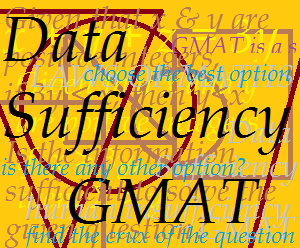## Solutions to GMAT DS Questions

Solution to Question in ‘Tackle Options in GMAT DS Questions the Oak’s Academy Way’ Blog PostQuestion:

Given that a, b, c, d, e are positive integers and that ‘b’ is an odd integer, is the product (a+b)(a+c)(a+d)(a+e) an odd integer?

(1) a is an odd integer

(2) c is an even integer

Solution:

In order to solve this question, we first need to review a few basic addition and multiplication rules:

 Addition Rules Multiplication Rules When Added Sum When Multiplied Product 1. odd + odd = even 4. odd × odd = odd 2. odd + even = odd 5. odd × even = even 3. even + even = even 6. even × even = even

Working with Statement (1)

Now according to the method we first take statement (1).

Statement (1) says, ‘a’ is odd and we already know that b is odd (the default information given in the question statement). Since odd + odd = even, the first bracket has to be even. Thus, the first bracket in the multiplication becomes even, and as soon as one number in a multiplication sum becomes even, the product HAS TO BE even (rules 5 and 6)!

Hence, statement (1) is sufficient to solve the question, so the answer has to be either (A) ‘Statement (1) alone is sufficient but statement (2) alone is not sufficient to answer the question asked’ or (D) ‘Each statement alone is sufficient to answer the question asked’’.

Working with Statement (2)

In order to decide whether the answer is (A) or (D), we go to the next step in the method: working with statement (2).

Statement (2) tells us that ‘c’ is even. We already know that ‘b is odd’ (the default information given in the question statement). But we have no information about whether ‘a’ is odd or even, so we have to consider both cases.

• Now, if ‘a’ is odd, the first bracket becomes even (since ‘b’ is odd, and odd + odd is even) and thus, the answer will also be EVEN.
• If ‘a’ is even, the first bracket becomes odd (since odd + even = odd). HOWEVER, there is one more thing we must note. Since ‘a’ and ‘c’ are even, the second bracket is even. Now, if even one number is even, the entire product has to be even (rule).

Thus, though information given in statement (2) apparently looks ambiguous and irrelevant, it is actually sufficient to reach a final answer!

Therefore, the answer is option (D)!